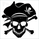จำนวนเข้าชม 23076
23076
Variable Moving Average, often abbreviated as VMA, is an Exponential Moving Average developed by Tushar S. Chande. VMA automatically adjusts its smoothing constant on the basis of Market Volatility .

Use this like other Moving Averages. I have added the following options that can be enabled via options page:
- Trend Direction Indication: Green = Up trend, Blue = Potential congestion, Red = down trend.
- Color bars based on Trend

List of my other indicators:
- Chart:
//
// @author LazyBear
// List of all my indicators:
//
study(title="Variable Moving Average [LazyBear]", shorttitle="VMA_LB", overlay=true)
src=close
l =input(6, title="VMA Length")
std=input(false, title="Show Trend Direction")
bc=input(false, title="Color bars based on Trend")
k = 1.0/l
pdm = max((src - src), 0)
mdm = max((src - src), 0)
pdmS = ((1 - k)*nz(pdmS) + k*pdm)
mdmS = ((1 - k)*nz(mdmS) + k*mdm)
s = pdmS + mdmS
pdi = pdmS/s
mdi = mdmS/s
pdiS = ((1 - k)*nz(pdiS) + k*pdi)
mdiS = ((1 - k)*nz(mdiS) + k*mdi)
d = abs(pdiS - mdiS)
s1 = pdiS + mdiS
iS = ((1 - k)*nz(iS) + k*d/s1)
hhv = highest(iS, l)
llv = lowest(iS, l)
d1 = hhv - llv
vI = (iS - llv)/d1
vma = (1 - k*vI)*nz(vma) + k*vI*src
vmaC=(vma > vma) ? green : (vma<vma) ? red : (vma==vma) ? blue : black
plot(vma, color=std?vmaC:black, linewidth=3, title="VMA")
barcolor(bc?vmaC:na)Close to the holey grail when combined with a MACD.
Would it be possible to add another Moving Average into the script like the Colored EMA SMA?
ตอบกลับMacChart
Is OK,... worked it out
Great MA!
ตอบกลับ